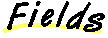`Packages  This Package  Prev  Next  Index  `

# §2.1 Class ColorModel

```public  abstract  class  java.awt.image.ColorModel
extends  java.lang.Object  (I-§1.12)
{
// Fields
protected int pixel_bits;	§2.1.1

// Constructors
public ColorModel(int  bits);	§2.1.2

// Methods
public abstract int getAlpha(int  pixel);	§2.1.3
public abstract int getBlue(int  pixel);	§2.1.4
public abstract int getGreen(int  pixel);	§2.1.5
public int getPixelSize();	§2.1.6
public abstract int getRed(int  pixel);	§2.1.7
public int getRGB(int  pixel);	§2.1.8
public static ColorModel getRGBdefault();	§2.1.9
}
```
This abstract class is the superclass for all classes that encapsulate methods for translating from pixel values to their alpha (transparency), red, green, and blue components.

The java.awt.image classes IndexColorModel (II-§2.6) and DirectColorModel (II-§2.3) are subclasses of this class.

##### pixel_bits

```protected int pixel_bits ```
The number of bits per pixel.

##### ColorModel

```public ColorModel(int bits) ```
Constructs a ColorModel which describes a pixel with the specified number of bits.
Parameters:
```bits - ```the number of bits per pixel

##### getAlpha

```public abstract int getAlpha(int pixel) ```
Determines the alpha transparency of a pixel in this color model. The value ranges from 0 to 255. The value 0 indicates that the pixel is completely transparent. The value 255 indicates that the pixel is opaque.
Parameters:
```pixel - ```a pixel value
Returns:
the alpha transparency represented by the pixel value.

### getBlue

```public abstract int getBlue(int pixel) ```
Determines the blue component of a pixel in this color model. The value ranges from 0 to 255. The value 0 indicates no contribution from this primary color. The value 255 indicates the maximum intensity of this color component.
Parameters:
```pixel - ```a pixel value
Returns:
the blue color component represented by the pixel value.

### getGreen

```public abstract int getGreen(int pixel) ```
Determines the green component of a pixel in this color model. The value ranges from 0 to 255. The value 0 indicates no contribution from this primary color. The value 255 indicates the maximum intensity of this color component.
Parameters:
```pixel - ```a pixel value
Returns:
The green color component ranging from 0 to 255

### getPixelSize

```public int getPixelSize() ```
Returns:
the number of bits per pixel in this color model.

### getRed

```public abstract int getRed(int pixel) ```
Determines the red component of a pixel in this color model. The value ranges from 0 to 255. The value 0 indicates no contribution from this primary color. The value 255 indicates the maximum intensity of this color component.
Parameters:
```pixel - ```a pixel value
Returns:
the red color component ranging from 0 to 255

### getRGB

```public int getRGB(int pixel) ```
Calculates a single integer representing the alpha, red, green, and blue components of a pixel in this color model. The components are each scaled to be a value between 0 and 255 . The integer returned is the number such that bits 24-31 are the alpha value, 16-23 are the red value, bits 8-15 are the green value, and bits 0-7 are the blue value.
Parameters:
```pixel - ```a pixel value
Returns:
an integer representing this color in RGB format.
getRGBdefault (II-§2.1.9).

### getRGBdefault

```public static ColorModel getRGBdefault() ```
Returns the default Abstract Window Toolkit color model.
The Abstract Window Toolkit represents each pixel as a 32-bit integer. Bits 24-31 are the alpha transparency, bits 16-23 are the red value, bits 8- 15 are the green value, and bits 0-7 are the blue value.
This method returns a ColorModel object which describes that pixel format and can be used to extract alpha, red, green, and blue values from such color values.
Returns:
the default Abstract Window Toolkit color model.

`Packages  This Package  Prev  Next  Index`
Java API Document (HTML generated by dkramer on April 22, 1996)